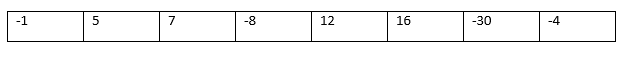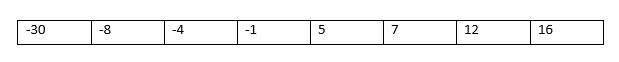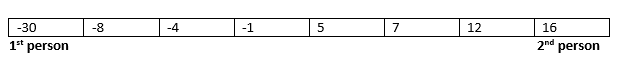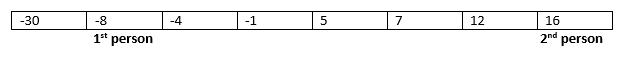# Find elements pair from array whose sum equal to number

Write a program to find a pair sum in array equal to given input number.

## Pair sum equal to a given number

[vc_row][vc_column width=”2/3″][td_block_text_with_title custom_title=”Method 1″][/td_block_text_with_title][/vc_column][/vc_row]

Time Complexity: O(NlogN)
Space Complexity: O(1)
Concept:

Let say there are numbers below and we need to find 2 numbers from an array whose sum is equal to 17

-1 5 7 -8 12 16 -30 -4And we need to find a pair whose sum is say 17

Below video explains the algorithm to find a pair of numbers from an array whose sum should be equal to given input number 17

Then the efficient way is shown below

1. Arrange the numbers in ascending order (Sort it )2. Run a loop this way :

• Let say one person stand at start of array and the second person stand at end• They add up the numbers on which they are standing=> -30 +16 =14
• If their number is equal to the given number X then they enjoy
• Else if the sum is lesser than the given number they ask the first person standing at start of array to move one step towards the end because if he travels right the number increases and hence the sum.• Similarly if the sum is more the person at the end is asked to move towards start by one step
• We repeat until the persons collide as after that the same pairs will be obtained.
• If no such pair is found then both become sad.
Important thing is the Sort() function.We should use Merge or Heap sort as they take O(NLogN) time.Or else we can use sort() STL function. with parameters “sort(arr,arr+size)”

Input:   -3 ,-4 , 10 , 0 ,3 ,-2 ,15 , 3
Sum:  7

Code for above algorithm in C++

```#include <bits/stdc++.h>
using namespace std;

int main()
{
int arr[] = {-3,-4,10,0,3,-2,15,3};
int size_of_array = sizeof(arr)/sizeof(arr);

//RequiredSum is number to which the pair should sum to

int RequiredSum = 7;

sort(arr,arr + size_of_array); //sort the array

int startIndex = 0 ,  endIndex = size_of_array - 1 , sum =0; //variables pointing on their respective indices and sum to store sum of the pair

while(startIndex <endIndex) //We require a pair so 2 elements and hence both elements should be of different indices
{
sum = arr[startIndex] + arr[endIndex];
if( sum == RequiredSum)
{
cout << "The numbers are " << arr[startIndex] <<" and " << arr[endIndex] <<endl;
return 0;
}

else if(sum < RequiredSum) //if sum is less then we need to increase the smaller one
startIndex ++;
else //if the sum if more we need to decrease the larger number
endIndex --;
}
cout << "No such pair exists.";
return 0;
}```

[vc_row][vc_column width=”2/3″][td_block_text_with_title custom_title=”Method 2″][/td_block_text_with_title][/vc_column][/vc_row]

Time Complexity – O(NlogN)
Space Complexity – O(N)
Concept:

Let say there are numbers in an array and we have to find the set of two elements from an array whose sum is equal to input number 23

-1 5 7 -8 12 16 -30 -4

and we need to find a pair whose sum is say 23

This concept is based on the Mapping approach to find elements pair from array whose sum equal to number

1. Create a map such that each element is added.
2. If there exists a pair that sums to X then both the elements are present in map.
3. So we loop through the array and do this

• Find if the (X – present) element is present in map
• If present then print the number.

You can use STL map data structure for this purpose.Or else you can create an array for indexing where index are the value of the array element itself.(But using array has a disadvantage that its size is required.)

EXPECTED

Input:   -3 ,-4 , 10 , 0 ,3 ,-2 ,15 , 3
Sum:  7

Code for above algorithm in C++

```#include <bits/stdc++.h>
using namespace std;

map <int,int> m;
map<int,int>::iterator it;

int main()
{
int arr[] = {-3,-4,10,0,3,-2,15,3};
int size_of_array = sizeof(arr)/sizeof(arr);

//cout << "Enter the number to which the pair should sum to"<<endl; //Let Sum = 7 int x = 7; //cin >> x; // the number to which the sum of pair of elements must be equal

int x = 7;
//cin >> x; // the number to which the sum of pair of elements must be equal

for (int i = 0; i < size_of_array;++i)
{
//Scan and add elements into the map
it = m.find(arr[i]);

if(it == m.end())
m.insert(make_pair(arr[i],1)); //Add the element in the map and set the count to 1 that represents it is present
}

for (int i = 0; i < size_of_array;i++)
{
it = m.find((x - arr[i]));  //If we have two numbers say m and n that sums to x then
//if we have m and if we find n in the map then we got the numbers.

if(it != m.end()) //If it exists then we got the pair
{
pair<int,int> p = *it; //Obtain the pair so as to reference the 2nd number
cout << "The numbers are " << arr[i] <<" and " << p.first <<endl;
return 0;
}
}

cout << "No such pair exists.";
return 0;
}```

Here we discussed two algorithms to find elements pair from array whose sum equal to number. This is a commonly asked question in technical interviews.

[vc_row][vc_column width=”2/3″][td_block_text_with_title custom_title=”Conclusion”][/td_block_text_with_title][/vc_column][/vc_row]

If interviewer is OK to use extra space then Method 2 is the best option to solve this question. But if he/she is not allowing you to use extra space for using Map then Method 1 is the best way to solve this question.

Translate »